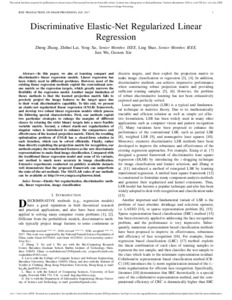# Discriminative elastic-net regularized linear regression

Zhang, Zheng, Lai, Zhihui, Xu, Yong, Shao, Ling, Wu, Jian and Xie, Guo-Sen (2017) Discriminative elastic-net regularized linear regression. IEEE Transactions on Image Processing, 26 (3). pp. 1466-1481. ISSN 1057-7149Preview PDF (Accepted manuscript) - Accepted Version Download (1MB) | Preview

## Abstract

In this paper, we aim at learning compact and discriminative linear regression models. Linear regression has been widely used in different problems. However, most of the existing linear regression methods exploit the conventional zeroone matrix as the regression targets, which greatly narrows the flexibility of the regression model. Another major limitation of theses methods is that the learned projection matrix fails to precisely project the image features to the target space due to their weak discriminative capability. To this end, we present an elastic-net regularized linear regression (ENLR) framework, and develop two robust linear regression models which possess the following special characteristics. First, our methods exploit two particular strategies to enlarge the margins of different classes by relaxing the strict binary targets into a more feasible variable matrix. Second, a robust elastic-net regularization of singular values is introduced to enhance the compactness and effectiveness of the learned projection matrix. Third, the resulting optimization problem of ENLR has a closed-form solution in each iteration, which can be solved efficiently. Finally, rather than directly exploiting the projection matrix for recognition, our methods employ the transformed features as the new discriminate representations to make final image classification. Compared with the traditional linear regression model and some of its variants, our method is much more accurate in image classification. Extensive experiments conducted on publicly available datasets well demonstrate that the proposed framework can outperform the state-of-the-art methods. The MATLAB codes of our methods can be available at http://www.yongxu.org/lunwen.html.

Item Type: Article image classification,elastic-net regularization,discriminative methods,linear regression Faculty of Science > School of Computing Sciences http://ieeexplore.ieee.org/document/7814... Pure Connector 18 Jan 2017 00:02 21 Oct 2022 08:32 https://ueaeprints.uea.ac.uk/id/eprint/62129 10.1109/TIP.2017.2651396View Item